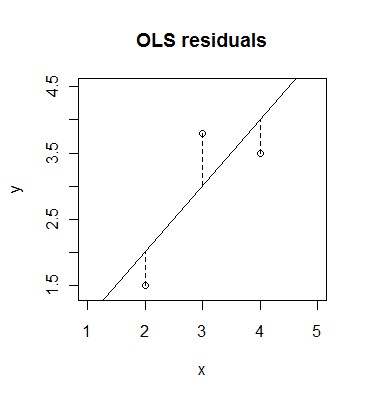# Total Least Squares

Statistical Analysis Techniques, Alternatives to OLSTotal least squares is an alternative regression method to the conventional ordinary least squares (OLS).  Total Least Squares is also known as errors in variables, rigorous least squares, and orthogonal regression.  It is a generalisation of Deming regression.

Total least squares is similar to OLS but instead of using vertical residuals (observed response value minus fitted response value), it uses diagonal residuals (shortest distant between observation point and fitted model).

The reason why total least squares isn’t used as often as ordinary least squares, is because problems arise when the variables have different units of measurement.  If the variables have different units of measurement and diagonal residual are used, then essentially quantities with different measurement units are being added together (due to Pythagoras Theorem).  To overcome this problem, the variables could be normalised / standardised.